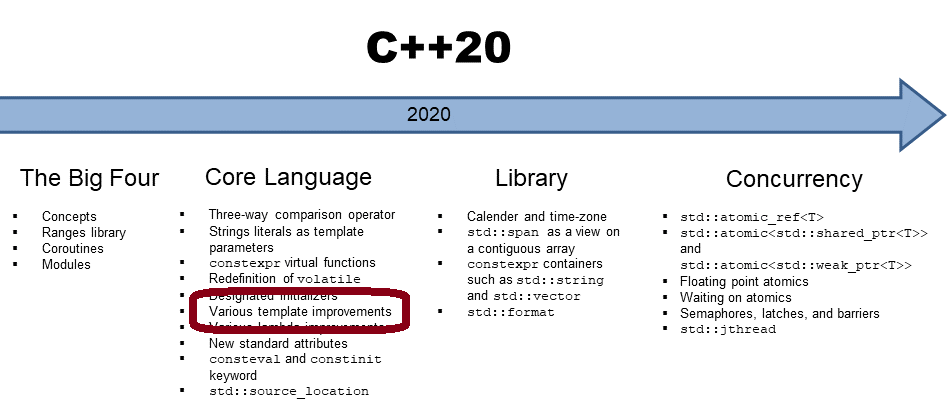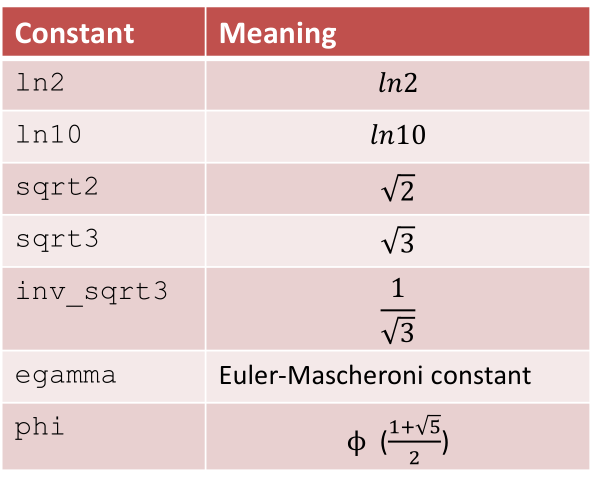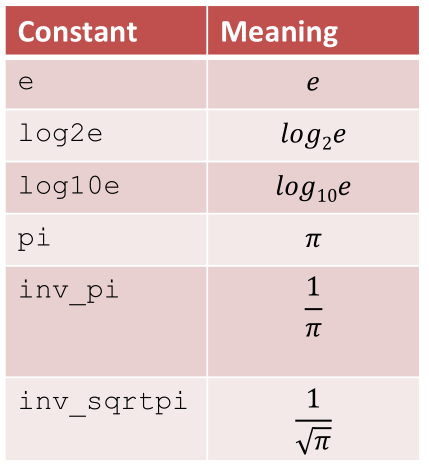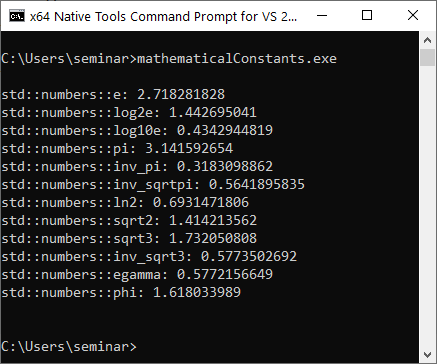# Safe Comparisons of Integrals with C++20

When you compare signed and unsigned integers, you may not get the result you expect. Thanks to the six `std::cmp_`* functions, there is a cure in C++20.Maybe, you remember the rule “ES.100 Don’t mix signed and unsigned arithmetic” from the C++ Core Guidelines. I wrote a few words about it in my previous post on “Arithmetic Rules“. Today, I want to investigate this issue and compare signed and unsigned integers.

## Unsafe Comparison of Integrals

Of course, there is a reason for the program name `unsafeComparison.cpp`.

##Modernes C++ Mentoring

Be part of my mentoring programs:

• "Fundamentals for C++ Professionals" (open)
• "Design Patterns and Architectural Patterns with C++" (open)
• "C++20: Get the Details" (reopens December 2023)
• Do you want to stay informed about my mentoring programs: Subscribe via E-Mail.

```// unsafeComparison.cpp

#include <iostream>

int main() {

std::cout << std::endl;

std::cout << std::boolalpha;

int x = -3;                  // (1)
unsigned int y = 7;          // (2)

std::cout << "-3 < 7:  " << (x < y) << std::endl;
std::cout << "-3 <= 7: " << (x <= y) << std::endl;
std::cout << "-3 > 7:  " << (x > y) << std::endl;
std::cout << "-3 => 7: " << (x >= y) << std::endl;

std::cout << std::endl;

}
```

When I execute the program, the output may not meet your expectations.When you read the program output, you recognize -3 should be bigger than 7. You presumably know the reason. I compared a `signed x` (line (1)) with an` unsigned y` (line (2)). What is happening under the hood? The following program provides the answer.

```// unsafeComparison2.cpp

int main() {
int x = -3;
unsigned int y = 7;

bool val = x < y;              // (1)
static_assert(static_cast<unsigned int>(-3) == 4'294'967'293);
}
```

In the example, I’m focusing on the less-than-operator. C++ Insights gives me the following output:Here is what’s happening:

1. The compiler transforms the expression` x < y `(line 1) into `static_cast<unsigned int>(x) < y`. In particular, the `signed` `x` is converted to an unsigned int.
2. Due to the conversion,` -3 `becomes 4’294’967’293.
3. ` 4'294'967'293` is equal to (-3) modulo (2 to the power of 32).
4. 32 is the number of bits of an unsigned` int` on C++ Insights.

Thanks to C++20, we have a safe comparison of integrals.

## Safe Comparison of Integrals

C++20 supports the six comparison functions for integrals:Thanks to the six comparison functions, I can easily transform the previous program` unsafeComparison.cpp` into the program `safeComparison.cpp. `The new comparison functions require the header `<utility`>.

```// safeComparison.cpp

#include <iostream>
#include <utility>

int main() {

std::cout << std::endl;

std::cout << std::boolalpha;

int x = -3;
unsigned int y = 7;

std::cout << "3 == 7:  " << std::cmp_equal(x, y) << std::endl;
std::cout << "3 != 7:  " << std::cmp_not_equal(x, y) << std::endl;
std::cout << "-3 < 7:  " << std::cmp_less(x, y) << std::endl;
std::cout << "-3 <= 7: " << std::cmp_less_equal(x, y) << std::endl;
std::cout << "-3 > 7:  " << std::cmp_greater(x, y) << std::endl;
std::cout << "-3 => 7: " << std::cmp_greater_equal(x, y) << std::endl;

std::cout << std::endl;

}
```

I also used in this program the equal and not equal operators.

Thanks to GCC 10, here is the expected result:Invoking a comparison function with a non-integral value would cause a compile-time error.

```// safeComparison2.cpp

#include <iostream>
#include <utility>

int main() {

double x = -3.5;             // (1)
unsigned int y = 7;          // (2)

std::cout << "-3.5 < 7:  " << std::cmp_less(x, y) << std::endl;

}
```

Trying to compare a `double` (line (1)) and an `unsigned int` (line (2)) gives the GCC 10 compiler a lengthy error message. Here is the crucial line of the error message:The internal type-traits  __is_standard_integer failed. I was curious about what that means and looked it up in the GCC type-traits implementation on GitHub. Here are the relevant lines from the header` type-traits:`

```// Check if a type is one of the signed or unsigned integer types.
template<typename _Tp>
using __is_standard_integer
= __or_<__is_signed_integer<_Tp>, __is_unsigned_integer<_Tp>>;

// Check if a type is one of the signed integer types.
template<typename _Tp>
using __is_signed_integer = __is_one_of<__remove_cv_t<_Tp>,
signed char, signed short, signed int, signed long,
signed long long

// Check if a type is one of the unsigned integer types.
template<typename _Tp>
using __is_unsigned_integer = __is_one_of<__remove_cv_t<_Tp>,
unsigned char, unsigned short, unsigned int, unsigned long,
unsigned long long
```

`__remove_cv_t` is the internal function of GCC to remove `const` or `volatile` from a type.

Maybe, you are now curious about what happens when you compare a `double` and an `unsigned int` the classical way.

Here is the modified program `safeComparison2.cpp.`

```// classicalComparison.cpp

int main() {

double x = -3.5;
unsigned int y = 7;

auto res = x < y;     // true

}
```

It works. The crucial `unsigned int` is floating-point promoted to `double`. C++ Insights shows the truth:After so many comparisons, I want to end this post with our new mathematical constants with C++20.

## Mathematical Constants

First, the constants require the header `<numbers>` and the namespace `std::numbers`. The following tables give you the first overview.The program `mathematicConstants.cpp` applies the mathematical constants.

```// mathematicConstants.cpp

#include <iomanip>
#include <iostream>
#include <numbers>

int main() {

std::cout << std::endl;

std::cout<< std::setprecision(10);

std::cout << "std::numbers::e: " <<  std::numbers::e << std::endl;
std::cout << "std::numbers::log2e: " <<  std::numbers::log2e << std::endl;
std::cout << "std::numbers::log10e: " <<  std::numbers::log10e << std::endl;
std::cout << "std::numbers::pi: " <<  std::numbers::pi << std::endl;
std::cout << "std::numbers::inv_pi: " <<  std::numbers::inv_pi << std::endl;
std::cout << "std::numbers::inv_sqrtpi: " <<  std::numbers::inv_sqrtpi << std::endl;
std::cout << "std::numbers::ln2: " <<  std::numbers::ln2 << std::endl;
std::cout << "std::numbers::sqrt2: " <<  std::numbers::sqrt2 << std::endl;
std::cout << "std::numbers::sqrt3: " <<  std::numbers::sqrt3 << std::endl;
std::cout << "std::numbers::inv_sqrt3: " <<  std::numbers::inv_sqrt3 << std::endl;
std::cout << "std::numbers::egamma: " <<  std::numbers::egamma << std::endl;
std::cout << "std::numbers::phi: " <<  std::numbers::phi << std::endl;

std::cout << std::endl;

}
```

Here is the output of the program with the MSVC compiler 19.27.The mathematical constants are available for `float`, `double`, and `long double`. Per-default `double` is used, but you can also specify `float` (`std::numbers::pi_v<float>`) or` long double` (`std::numbers::pi_v<long double>`).

## What’s next?

C++20 offers more valuable utilities. For example, you can ask your compiler which C++ feature it supports, and can easily create functional objects with `std::bind_front, `or perform different actions in a function whether the function runs a compile-time or at runtime.

Thanks a lot to my Patreon Supporters: Matt Braun, Roman Postanciuc, Tobias Zindl, G Prvulovic, Reinhold Dröge, Abernitzke, Frank Grimm, Sakib, Broeserl, António Pina, Sergey Agafyin, Андрей Бурмистров, Jake, GS, Lawton Shoemake, Jozo Leko, John Breland, Venkat Nandam, Jose Francisco, Douglas Tinkham, Kuchlong Kuchlong, Robert Blanch, Truels Wissneth, Kris Kafka, Mario Luoni, Friedrich Huber, lennonli, Pramod Tikare Muralidhara, Peter Ware, Daniel Hufschläger, Alessandro Pezzato, Bob Perry, Satish Vangipuram, Andi Ireland, Richard Ohnemus, Michael Dunsky, Leo Goodstadt, John Wiederhirn, Yacob Cohen-Arazi, Florian Tischler, Robin Furness, Michael Young, Holger Detering, Bernd Mühlhaus, Matthieu Bolt, Stephen Kelley, Kyle Dean, Tusar Palauri, Dmitry Farberov, Juan Dent, George Liao, Daniel Ceperley, Jon T Hess, Stephen Totten, Wolfgang Fütterer, Matthias Grün, Phillip Diekmann, Ben Atakora, Ann Shatoff, Rob North, Bhavith C Achar, and Marco Parri Empoli.

Thanks, in particular, to Jon Hess, Lakshman, Christian Wittenhorst, Sherhy Pyton, Dendi Suhubdy, Sudhakar Belagurusamy, Richard Sargeant, Rusty Fleming, John Nebel, Mipko, Alicja Kaminska, Slavko Radman, and David Poole.

 My special thanks to EmbarcaderoMy special thanks to PVS-StudioMy special thanks to Tipi.buildMy special thanks to Take Up Code## Seminars

I’m happy to give online seminars or face-to-face seminars worldwide. Please call me if you have any questions.

### Standard Seminars (English/German)

Here is a compilation of my standard seminars. These seminars are only meant to give you a first orientation.

• C++ – The Core Language
• C++ – The Standard Library
• C++ – Compact
• C++11 and C++14
• Concurrency with Modern C++
• Design Pattern and Architectural Pattern with C++
• Embedded Programming with Modern C++
• Generic Programming (Templates) with C++

#### New

• Clean Code with Modern C++
• C++20

### Contact Me

Modernes C++ Mentoring,0 replies